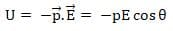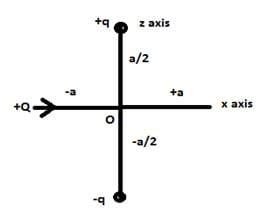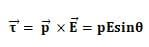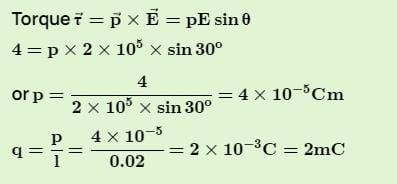NEET  >  Test: Electric Dipole

# Test: Electric Dipole

Test Description

## 10 Questions MCQ Test Physics Class 12 | Test: Electric Dipole

Test: Electric Dipole for NEET 2023 is part of Physics Class 12 preparation. The Test: Electric Dipole questions and answers have been prepared according to the NEET exam syllabus.The Test: Electric Dipole MCQs are made for NEET 2023 Exam. Find important definitions, questions, notes, meanings, examples, exercises, MCQs and online tests for Test: Electric Dipole below.
Solutions of Test: Electric Dipole questions in English are available as part of our Physics Class 12 for NEET & Test: Electric Dipole solutions in Hindi for Physics Class 12 course. Download more important topics, notes, lectures and mock test series for NEET Exam by signing up for free. Attempt Test: Electric Dipole | 10 questions in 10 minutes | Mock test for NEET preparation | Free important questions MCQ to study Physics Class 12 for NEET Exam | Download free PDF with solutions
 1 Crore+ students have signed up on EduRev. Have you?
Test: Electric Dipole - Question 1

### Which of the following molecule has permanent dipole moment?​

Detailed Solution for Test: Electric Dipole - Question 1

Ionic behavior implies dipole moment.

HCl is an ionic compound (H+Cl-)
CO2, CH4 and BF3  is a covalent compound

Test: Electric Dipole - Question 2

### A dipole is said to be in stable equilibrium when angle between electric field and dipole moment is:​

Detailed Solution for Test: Electric Dipole - Question 2Stable equilibrium is attained when potential energy of the dipole system is minimum which is possible at an inclination of 0°.

Test: Electric Dipole - Question 3

### What is the angle between the electric dipole moment and the electric field on the equatorial line of the dipole?

Detailed Solution for Test: Electric Dipole - Question 3

On equatorial line, the direction of electric field is reversed to that of angle of axial line. Therefore, the angle between dipole moment and electric field is 180°.

Test: Electric Dipole - Question 4

An electric dipole is

Detailed Solution for Test: Electric Dipole - Question 4

An electric dipole is a separation of positive and negative charges. The simplest example of this is a pair of electric charges of equal magnitude but opposite sign, separated by some (usually small) distance. A permanent electric dipole is called an electret.

Test: Electric Dipole - Question 5

How does electric field strength change with distance from a short dipole?

Detailed Solution for Test: Electric Dipole - Question 5

Electric Field Intensity due to a short dipole is given by

E = K2P/r^3

where K is the proportionality constant

P is the electric dipole moment

So electric field intensity is inversely proportional to r^3

Test: Electric Dipole - Question 6

An electric dipole consists of a positive and negative charge of 4 µC. Each placed at a distance of 5 mm. The dipole moment for the system is

Detailed Solution for Test: Electric Dipole - Question 6

q= 4μc = 4 x 10-6c
2l = 5mm = 5 x 10-3m
Dipole moment  P = q.2l
= 4 x 10-6 x 5 x 10-3
= 20 x 10-9
= 2 x 10 x 10-9
= 2 x 10-8 c.m

its direction is toward the positive charge.

Test: Electric Dipole - Question 7

Which of the following quantity is a scalar?

Detailed Solution for Test: Electric Dipole - Question 7

Electric flux is a scalar quantity. It is a scalar because it is the dot product of two vector quantities, electric field and the perpendicular differential area.

Test: Electric Dipole - Question 8

For forming an electric dipole between two point charges separated by a small distance, the two point charges are of

Detailed Solution for Test: Electric Dipole - Question 8

By definition of Electric Dipole

Test: Electric Dipole - Question 9

Positive and negative point charges of equal magnitude are kept at locations ( 0,0,a/2) and (0,0,-a/2) respectively. The work done by the electric field when another positive point charge is moved from (-a,0,0) to (0,a,0) is

Detailed Solution for Test: Electric Dipole - Question 9+Q charge is moving on equatorial line of dipole as shown in figure, since on equatorial line V=0,
Hence the potential difference is zero. So, W=0

Test: Electric Dipole - Question 10

An electric dipole is placed at an angle of 30° with an electric field intensity 2 × 105 N/C. It experiences a torque equal to 4 N m. The charge on the dipole, if the dipole length is 2 cm, is

Detailed Solution for Test: Electric Dipole - Question 10

Torque on a dipole kept in external electric field is given by the expression,## Physics Class 12

157 videos|452 docs|213 tests
 Use Code STAYHOME200 and get INR 200 additional OFF Use Coupon Code
Information about Test: Electric Dipole Page
In this test you can find the Exam questions for Test: Electric Dipole solved & explained in the simplest way possible. Besides giving Questions and answers for Test: Electric Dipole, EduRev gives you an ample number of Online tests for practice

## Physics Class 12

157 videos|452 docs|213 tests

### How to Prepare for NEET

Read our guide to prepare for NEET which is created by Toppers & the best Teachers